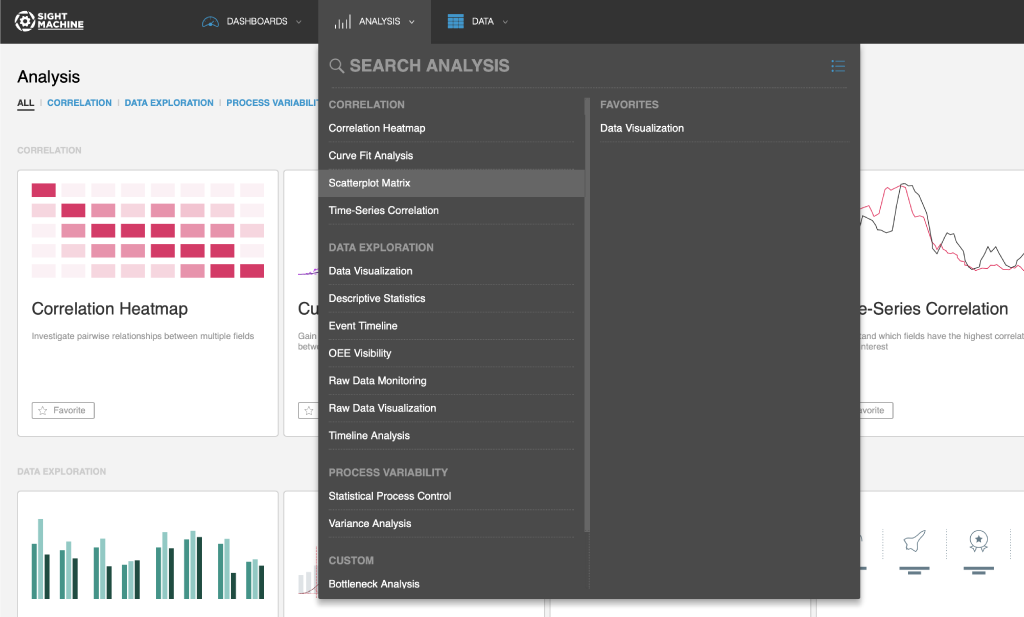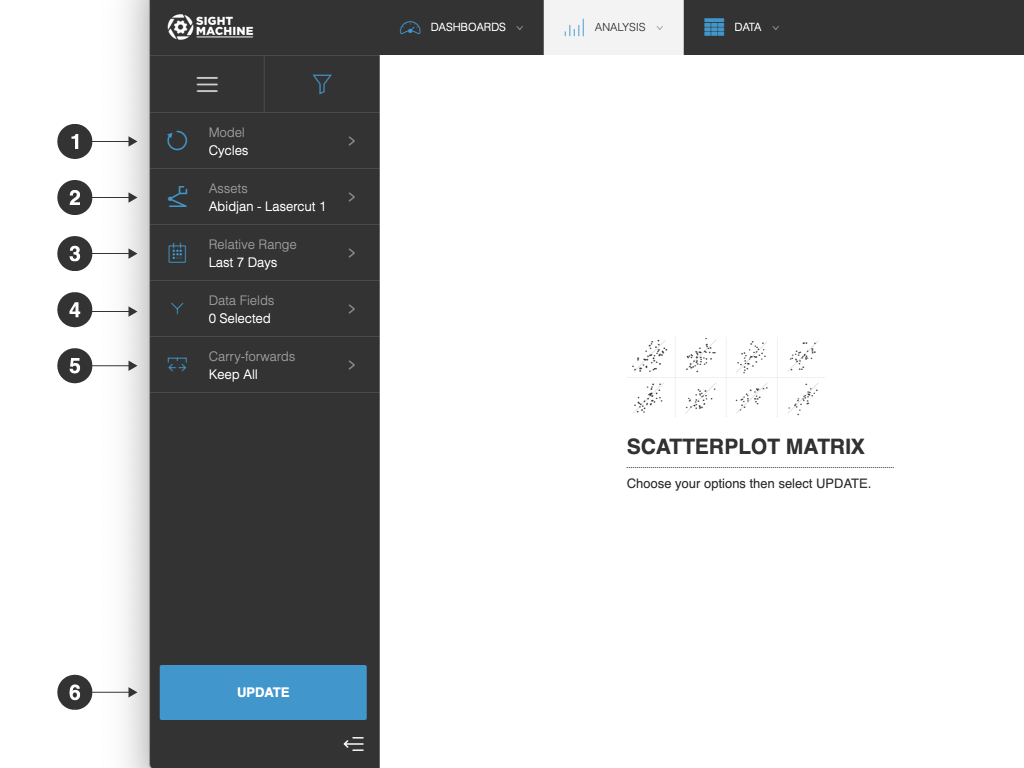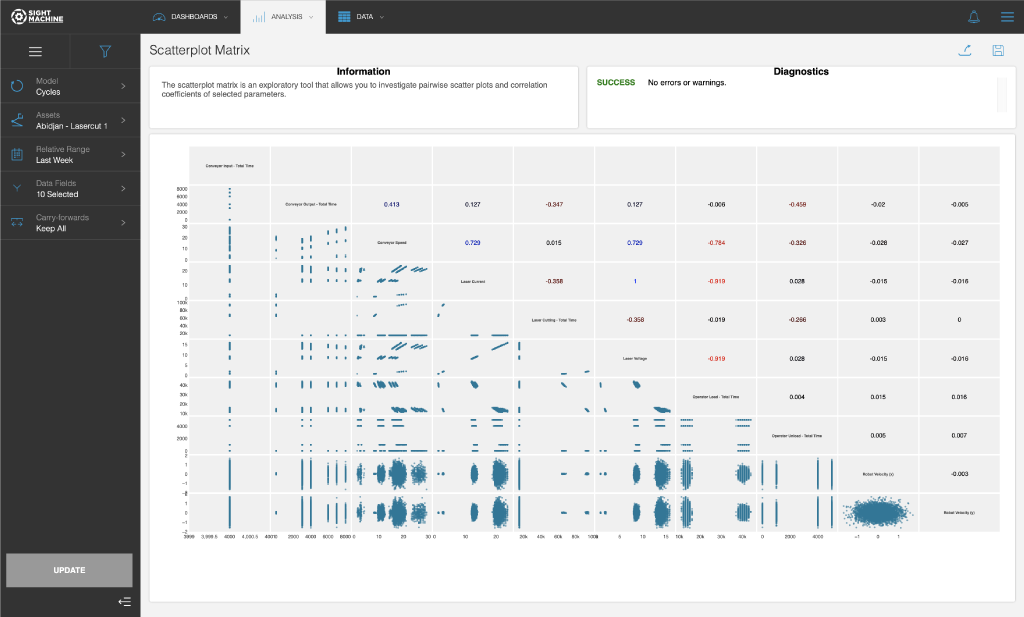# Use Cases

The Scatterplot Matrix plots the pairwise scatterplot for all combinations of the selected parameters. It is designed for comparing multiple parameters at the same time, with a greater level of detail than the Correlation Heatmap and with more breadth than the Curve Fit Analysis. This tool is most helpful early in the exploratory process to identify patterns that you want to investigate in more detail. This is a good tool to lead to Curve Fit Analysis.

# Specifications

The Scatterplot Matrix is a multivariate analysis that you can perform on continuous fields on the Cycle and Part models. You can select multiple machines of a given type when the analysis is performed on the Cycle model. Using the Cycle model, the Scatterplot Matrix can investigate parameters from the same machine type with high granularity. Using the Part model lets you compare fields across different machines, but with lower granularity (the Part model is limited by the serial number or other identifier used to construct the data model).

# Analysis

The Scatterplot Matrix allows you to investigate the pairwise relationships among up to 10 parameters at the same time. The downward diagonal in the matrix labels the parameters. For example, the lower-left corner plot shown is Conveyor Speed (X axis) vs. Laser Current (Y axis). The lower-left side of the matrix plots the scatterplot for each parameter combination. The upper-right side of the matrix gives the Pearson-R correlation coefficient for each pair.

# How to Use a Scatterplot Matrix

The Scatterplot Matrix lets you analyze continuous fields on the Cycle and Part models.

To use a Scatterplot Matrix:

1. On the Analysis tab, under Correlation, click Scatterplot Matrix.2. On the main Scatterplot Matrix screen, select your options on the left. For more details about each option, see Scatterplot Matrix Options.
3. Click Update.

# Scatterplot Matrix OptionsThe Scatterplot Matrix options include:

1.  Model: You can select:
• Cycles: Examine the correlation between two parameters in the same asset or asset type.
• Parts: Examine the correlation between two parameters across different machines producing the same part type.
2. Assets: You can select assets to monitor. For example, to perform a Cycles analysis, you need to select an asset to analyze. You can select 2 specific machines. If you are using the Parts model, you need to select a part type.
3. Relative Range: You can define a date range of data points to analyze for the assets you have selected. For example, select the last 7 days. This option establishes the boundaries for the near real-time data that will be part of your analysis. Select from relative or absolute timeframes.
4. Data Fields: You can select data fields to analyze.
5. Carry-forwards: You can undo the forward-fill effect on any fields. Select from Keep All, First, or Last. For more information, see the table in Options Common to All Analysis Tools.
6. Update: Click this button to generate your chart.# Tool Output

The Scatterplot Matrix returns a matrix of scatterplots and correlation coefficients. Each cell below the diagonal contains a scatterplot comparing the two relevant fields. Each cell above the diagonal contains the correlation coefficient for those two fields.